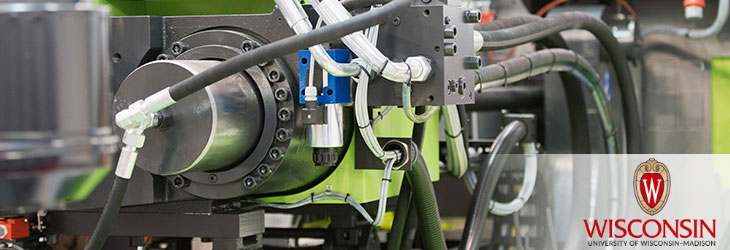Wisconsin Alumni Research Foundation

# WARF TECHNOLOGIES

EngineeringHigh Force Density Three Pole Magnetic Bearing
WARF: P190159US01

Inventors: Eric Severson, Nicholas Hemenway

The Invention
A first radial force value and a second radial force value is received by a radial magnetic bearing controller. Coefficients are computed for a first equation using the first and second radial force values. The first equation is solved to define first solution values. A second solution value paired with each first solution value is computed using the first radial force value and a respective first solution value to define second solution values. Control current sets are computed for each unique paired solution of the second solution values and the first solution values. Each control current set includes a control current value for each of three control currents. A control current value for each of the three control currents is selected from the control current sets. The control current value for each of the three control currents is output to a respective radial winding of a three-pole radial magnetic bearing.